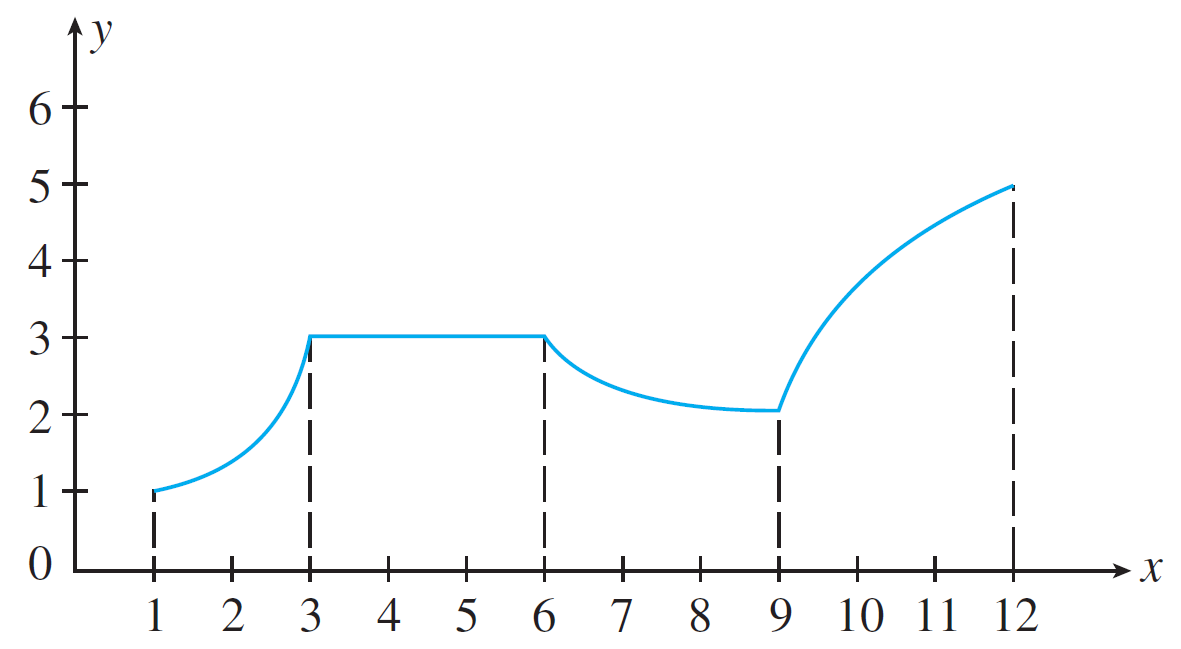# Homework 8

Directions:

1. Show each step of your work and fully simplify each expression.
2. Turn in your answers in class on a physical piece of paper.
3. Staple multiple sheets together.
4. Feel free to use Desmos for graphing.

1. Given the following function:Find the sign of each of the following:
1. $f'(x)$ on $(1, 3)$
2. $f'(4)$
3. $f'(6)$
4. $f'(x)$ on $(6, 9)$
5. $f'(12)$ (hint: derivative is a limit. when do limits exist?)
6. $f'(x)$ on $(9, 12)$
2. Again consider the function above. Determine the critical numbers and give the reason why they are a critical number.
3. Find the intervals where each function is increasing/decreasing and their relative maxima/minima, if they exist. Use methods described in class.
1. $f(x) = x - x^3$
2. $f(x) = x^4 - 4x^3 + 10$
3. $f(x) = \dfrac{x + 1}{x}$
4. $f(x) = \dfrac{x^2}{x + 1}$
5. $f(x) = x\sqrt{x + 1}$
4. The height of a rocket in meters follows the polynomial function $f(t) = -\dfrac{1}{3}t^3 + 16t^2 + 33t + 10$ where $t$ represents $t$ seconds from launch. Determine which seconds the rocket is rising and falling.